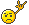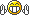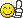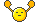Novice: Trenutno ni obvestil.
Dobrodošel, Gost. Prosim, prijavi se ali se registriraj.

ZEVS ForumVremeSplošnoRazlage in enačbe parametrov in indeksov vertikalnih sondaž
 Strani:Avtor Tema: Razlage in enačbe parametrov in indeksov vertikalnih sondaž  (Prebrano 2173 krat) 0 Člani in 1 Gost gledajo to temo.
Ciklon
StratokumulusOdsoten

RD: 1987-06-24
Sporočila: 3108Lokacija: Voglje, 375m n.v., cca 2 km zračne razdalje od letališča J. Pučnika (na J strani avtoceste)« dne: 11. April 2009, 20:12:01 »

Sounding Station Parameters and Indices

SLAT   Station latitude in degrees

SLON   Station longitude in degrees; West longitude is negative

SELV   Station elevation in meters

SHOW   Showalter index
SHOW   = T500 - Tparcel
T500   = Temperature in Celsius at 500 mb
Tparcel   = Temperature in Celsius at 500 mb of a parcel lifted from 850 mb

LIFT   Lifted index
LIFT   = T500 - Tparcel
T500   = temperature in Celsius of the environment at 500 mb
Tparcel   = 500 mb temperature in Celsius of a lifted parcel with the average pressure, temperature, and dewpoint of the layer 500 m above the surface

LFTV   LIFT computed by using virtual temperature.

SWET   SWEAT index
SWET   = 12 * TD850 + 20 * TERM2 + 2 * SKT850 + SKT500 + SHEAR
TD850    = Dewpoint in Celsius at 850 mb
TERM2    = MAX ( TOTL - 49, 0 )
TOTL    = Total totals index
SKT850   = 850 mb wind speed in knots
SKT500   = 500 mb wind speed in knots
SHEAR    = 125 * [ SIN ( DIR500 - DIR850 ) + .2 ]
DIR500   = 500 mb wind direction
DIR850   = 850 mb wind direction

KINX   K index
KINX   = ( T850 - T500 ) + TD850 - ( T700 - TD700 )
T850    = Temperature in Celsius at 850 mb
T500    = Temperature in Celsius at 500 mb
TD850    = Dewpoint in Celsius at 850 mb
T700    = Temperature in Celsius at 700 mb
TD700    = Dewpoint in Celsius at 700 mb

CTOT   Cross Totals index
CTOT   = TD850 - T500
TD850    = Dewpoint in Celsius at 850 mb
T500    = Temperature in Celsius at 500 mb

VTOT   Vertical Totals index
VTOT   = T850 - T500
T850   = Temperature in Celsius at 850 mb
T500   = Temperature in Celsius at 500 mb

TOTL    Total Totals index
TOTL   = ( T850 - T500 ) + ( TD850 - T500 )
T850    = Temperature in Celsius at 850 mb
TD850   = Dewpoint in Celsius at 850 mb
T500    = Temperature in Celsius at 500 mb

CAPE   Convective Available Potential Energy (J/kg)
CAPE   = GRAVTY * SUMP ( DELZ * ( TP - TE ) / TE )
SUMP   = sum over sounding layers from LFCT to EQLV for which ( TP - TE ) is greater than zero
DELZ   = incremental depth
TP    = temperature of a parcel from the lowest 500 m of the atmosphere, raised dry adiabatically to the LCL and moist adiabatically thereafter
TE    = temperature of the environment

CAPV   CAPE computed by using virtual temperature.
CAPV   = GRAVTY * SUMP ( DELZ * ( TVP - TVE ) / TVE )
SUMP   = sum over sounding layers from LFCV to EQTV for which ( TVP - TVE ) is greater than zero
DELZ   = incremental depth
TVP    = virtual temperature. of a parcel from the lowest 500 m of the atmosphere, raised dry adiabatically to the LCL and moist adiabatically thereafter
TVE    = virtual temperature. of the environment

CINS   Convective Inhibition (J/kg)
CINS   = GRAVTY * SUMN ( DELZ * ( TP - TE ) / TE )
SUMN   = sum over sounding layers from top of the mixed layer to LFCT for which ( TP - TE ) is less than zero.
DELZ   = incremental depth
TP    = temperature of a parcel from the lowest 500 m of the atmosphere, raised dry adiabatically to the LCL and moist adiabatically thereafter
TE    = temperature of the environment

CINV   CINS computed by using virtual temperature.
CINV   = GRAVTY * SUMN ( DELZ * ( TVP - TVE ) / TVE )
SUMN   = sum over sounding layers from top of the mixed layer to LFCV for which ( TVP - TVE ) is less than zero.
DELZ   = incremental depth
TVP    = virtual temperature. of a parcel from the lowest 500 m of the atmosphere, raised dry adiabatically to the LCL and moist adiabatically thereafter
TVE    = virtual temperature. of the environment

EQLV    Equilibrium level (hPa)
EQLV   = level at which a parcel from the lowest 500 m of the atmosphere is raised dry adiabatically to the LCL and moist adiabatically to a level above which the temperature of the parcel is the same as the environment. If more than one Equilibrium Level exists, the highest one is chosen.

EQTV   EQLV computed by using virtual temperature.

LFCT   Level of Free Convection (hPa) by comparing temperature between a parcel and the environment

LFCT   = level at which a parcel from the lowest 500 m of the atmosphere is raised dry adiabatically to LCL and moist adiabatically to the level above which the parcel is positively buoyant. If more than one LFCT exists, the lowest level is chosen. If the parcel is positively bouyant throughout the sounding, the LFCT is set to be the same as the LCLP.
LFCV   LFCT computed by using virtual temperature.

BRCH   Bulk Richardson number
BRCH   = CAPE / ( 0.5 * U**2 )
CAPE    = Convective Available Potential Energy
U    = magnitude of shear ( u2 - u1, v2 - v1 )
u1,v1   = average u,v in the lowest 500 m
u2,v2   = average u,v in the lowest 6000 m

BRCV   BRCH computed by using CAPV
BRCV   = CAPV / ( 0.5 * U**2 )
CAPV    = CAPE computed by using virtual temperature.
U    = magnitude of shear ( u2 - u1, v2 - v1 )
u1,v1   = average u,v in the lowest 500 m
u2,v2   = average u,v in the lowest 6000 m

LCLT   Temperature (K) at the LCL, lifted condensation level, from an average of the lowest 500 meters.
LCLT   = [1 / ( 1 / ( DWPK - 56 ) + LN ( TMPK / DWPK ) / 800 )] + 56

LCLP   Pressure (hPa) at the LCL, lifted condensation level, from an average of the lowest 500 meters.
LCLP   = PRES * ( LCLT / ( TMPC + 273.15 ) ) ** ( 1 / KAPPA )

Poisson's equation

MLTH   Mean mixed layer THTA (K)
MLTH   = average THTA in the lowest 500 m

MLMR   Mean mixed layer MIXR (g/kg)
MLMR   = average MIXR in the lowest 500 m

THTK   1000 mb to 500 mb thickness (meter)
THTK   = ( Z500 - Z1000 )
Z500    = Height of the 500 mb surface
Z1000   = Height of the 1000 mb surface

PWAT   Precipitable water (mm) for the entire sounding

vir: http://weather.uwyo.edu/
 « Zadnje urejanje: 11. April 2009, 20:13:43 od Ciklon »Prijavljen

Raj je v naraviEkolog
Gost« Odgovori #1 dne: 11. April 2009, 21:00:11 »

Super razlage, čestitke za trudPrijavljen
 Strani: 
Pojdi na: